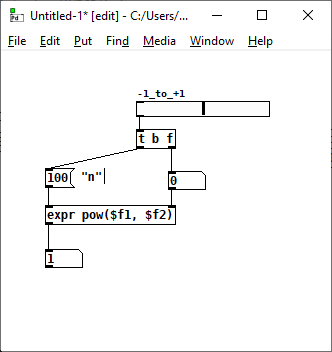• ### Sliders and linear to log scaling behavior

I'm trying to solve the maths behind the lin to log conversion in the sliders properties:

• Give a log slider a (0.1-10) range, and its centre position will be equal to 1
• Give a log slider a (0.01-100) range, and its centre position will still be 1

This is a great behavior for pitch scaling when you want to create a frequency offset of an oscillator in relation to another. For instance, with a (0.1-10) range, you get unison at centre position, +10 octaves at max position and -10 octaves at min position.

I want to recreate a similar behavior by converting a positive signal ranging (0 to n) linearly to a signal ranging (1/n - n) logarithmically.

The function should be something like this I believe:
f(0) = 1/n
f(n/2) = 1
f(n) = n

Did a bit of research and I think the function should look something like: y = a exp (bx).

My maths is a bit rusty I'm afraid. Could you help me solve this problem?

• Posts 3 | Views 864
• @tomatoKetchup
n raised to -1 equals 1/n
n raised to 0 equals 1
n raised to 1 equals n
So your problem is to map numbers in the domain 0...n to the range -1...1 and then raise n to that power.

(Let me know if that was too vagueI'm assuming you're like me in that you enjoy figuring stuff out for yourself, but it's OK if I'm wrong)

• ``````n raised to -1 equals 1/n
n raised to 0 equals 1
n raised to 1 equals n
``````

@jameslo Of course!!! The answer was so obvious, how did I miss it! Told you my maths was rusty as hell!Here's the answer, for those interested but in worth arithmetical condition than me...Posts 3 | Views 864
Internal error.

Oops! Looks like something went wrong!